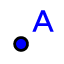# GeoGebra 3D Beginner Tutorial: Exercise 1

## NEW TOOLS WE WILL LEARN HOW TO USE:

MovePointPlane Through 3 PointsIntersect Two SurfacesIn this introductory exercise, we'll learn a means through which students can discover the intersection of any 2 (non-parallel) planes.

## DIRECTIONS:

1) Select the POINTtool. Use this tool to plot 3 points anywhere on the gray plane. 2) Select the MOVEarrow. Click twice on any one of the points you plotted in step (1).Use this tool to move this point off of the gray plane. Repeat this process for the other 2 points. 3) Select the PLANE THROUGH 3 POINTStool. With this tool highlighted, select the 3 points you initially plotted in step (1). This will create a plane that passes through these 3 points. Note: This step could have also been done without plotting the points first (step 1). Steps (1) - (2) were included here simply to acquaint you with plotting & moving points in 3D. Further directions appear below this applet.
4) Select the MOVEarrow. Use this tool to drag any one (or more) of these 3 points around. Notice how the plane you plotted in (3) always passes through these 3 points. 5) Now select the INTERSECT 2 SURFACEStool. Select the plane you plotted in step (3). Then select the gray plane. This will now plot the intersection of these 2 surfaces (here, planes).

## QUESTION:

What can we conclude about the intersection of any 2 non-parallel planes?

When you're done (or if you're unsure of something), feel free to check by watching the quick silent screencast below the applet.